# Constructing a Bisector of an angle:

Take a sheet of paper. Mark a point O on it. With O as the initial point, draw two rays bar"OA" and bar"OB". You get ∠ AOB. Fold the sheet through O such that the rays bar"OA" and bar"OB" coincide.
Let OC be the crease of paper which is obtained after unfolding the paper. OC is clearly a line of symmetry for ∠AOB.

OC the line of symmetry is therefore known as the angle bisector of ∠AOB.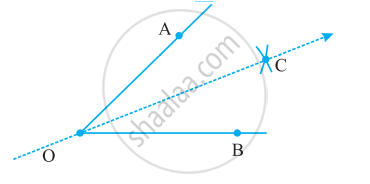## Construction with ruler and compasses:

Let an angle, say, ∠A be given.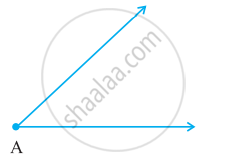Step 1:  With A as a centre and using compasses, draw an arc that cuts both rays of ∠ A. Label the points of intersection as B and C.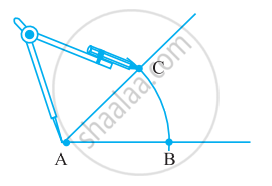Step 2: With B as the centre, draw (in the interior of ∠A ) an arc whose radius is more than half the length BC.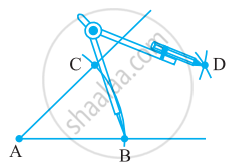Step 3: With the same radius and with C as a centre, draw another arc in the interior of ∠A. Let the two arcs intersect at D. Then bar"AD" is the required bisector of ∠ A.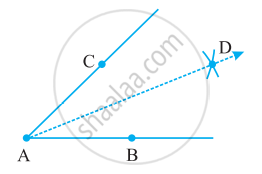If you would like to contribute notes or other learning material, please submit them using the button below.

### Shaalaa.com

Constructing a Bisector of an angle [00:02:11]
S
0%